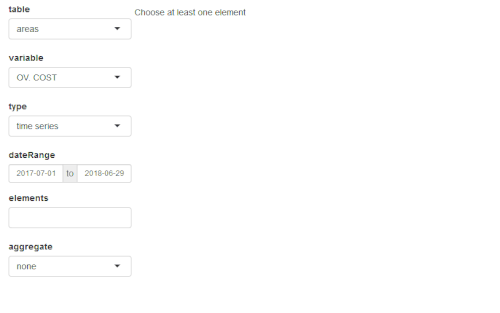# Quick presentation of antaresViz

#### 2021-11-24

This package works along with RTE’s adequacy software ANTARES : https://antares-simulator.org/

`antaresViz` is a package which proposes relevant graphs and maps to vizualize the results of an ANTARES study, with numerous settings and possible customizations.

## Where everything starts

``library(antaresViz)``

The data from an ANTARES study can be imported and easily manipulated using the antaresRead package.

The examples presented below have been build on a fictionnal study whose output have been loaded with antaresRead. They contains different types of elements :

• outputs with an annual and an hourly time step,
• synthetic outputs (averaged on several Monte-Carlo years) and detailled outputs (given year by year),
• outputs for areas, links, clusters and districts.
``load("data_for_antaresViz_vignette_extralight.Rdata")``

The aim of this vignette is to give a quick overview of the possibilities offered by the package antaresViz. The data with which the following graphs have been plotted are fictionnal.

## The simple power of the `plot` function

The `plot()` function, used with an antaresDataList object (i.e. an object returned by the `antaresRead::readAntares()` function) offers different possible vizualisations.

Moreover, this function is really easy to use. It opens a shiny interface which let the user decide :

• Which type of element he wants to study : areas, links or disctricts.
• Which variable he wants to analyze.
• With which type of graph : barplot, time series, probability density function, heatmap, etc.
• For which area(s), link(s), or district(s).
• And during which time period.
``plot(data_hourly_synthesis)``Note that all the interface manipulation can also be set directly in the arguments of the `plot` function. Some examples of the multiple graphs that can be returned by this function are given below.

###### Barplot of Loss of Load Duration (LOLD) for several districts of the study
``````plot(
data_annual_synthesis,
table = "districts",
variable = "LOLD",
type = "barplot",
elements = c("00_a", "00_b", "00_c", "00_d",
"00_e", "00_f", "00_g", "00_h", "00_i"),
interactive = FALSE,
width = "100%",
height = 400
)``````
###### Time series of the load in an area with average value and 95% confidence interval.
``````plot(
data_hourly_allmc,
table = "areas",
type = "ts",
elements = "23_b",
confInt = 0.95,
dateRange = c("2018-01-08", "2018-01-14"),
width = "100%",
height = 400,
interactive = FALSE
)``````
###### Probability density function of the wind power generation in two areas
``````plot(
data_hourly_synthesis,
table = "areas",
variable = "WIND",
type = "density",
elements = c("01_a", "02_a"),
interactive = FALSE,
width = "100%",
height = 400
)``````
###### Heatmap of the congestion probability of one interconnection
``````plot(
data_hourly_synthesis_1year,
variable = "CONG. PROB +",
type = "heatmap",
elements = "25_c - 26_d",
interactive = FALSE,
width = "100%",
height = 400,
main = "Congestion probability"
)``````

Note that the `plot()` function also contains a `compare` argument which allows comparisons between :

• different variables
• different studies

## When the production meets the demand

The `prodStack` function builds a graph which contains the time series of demand in one area (or the sum of the demand of a set of areas) along with the generation of this area (or set of areas), divided between the different fuel types (e.g. nuclear, gas, wind, etc).

``prodStack(data_hourly_synthesis)``

Once again, this function is easy to use and opens a shiny interface which let the user manipulate the selected areas and time range. Some settings can also be passed to the function through its (optionnal) arguments.

``````prodStack(
data_hourly_synthesis,
stack = "eco2mix",
areas = "37_h",
dateRange = c("2018-01-08", "2018-01-21"),
main = "Production stack",
unit = "GWh",
interactive = FALSE,
width = "100%",
height = 500
)``````

The graphical template used by default is the one of the RTE’s application eco2mix. This template can though be redefined completely by the user with the function `setProdStackAlias()`.

The `exchangesStack()` function proposes similar graphs with a superposition of all the imports and exports of an area.

``````exchangesStack(
data_hourly_synthesis,
area = "37_h",
dateRange = c("2018-01-08", "2018-01-21"),
main = "Import/Export of area 37_h",
unit = "GWh",
interactive = FALSE,
width = "100%",
height = 500
)``````

## Everything looks better on a map

Last but not least, `antaresViz` proposes several function to vizualise the results of a study on a map.

To do so, the first function to use is `mapLayout()`. This function launches an interactive application that let the user place areas of the ANTARES study on a map.

``````antares_layout <- antaresRead::readLayout(opts = antaresRead::setSimulationPath(study_path))
map_layout <- mapLayout(layout = antares_layout)``````